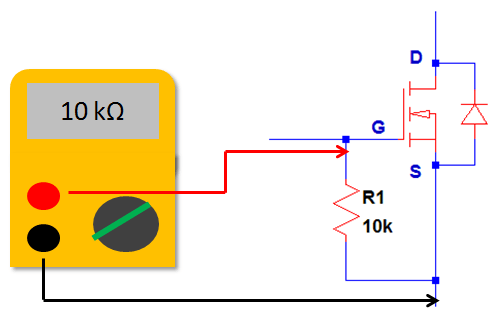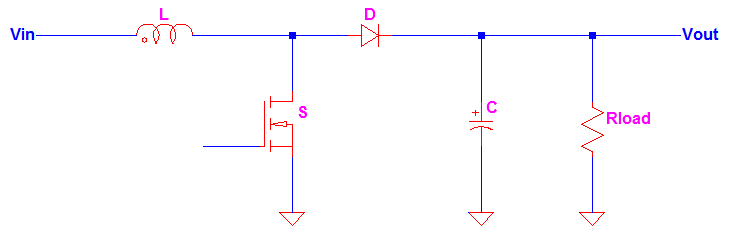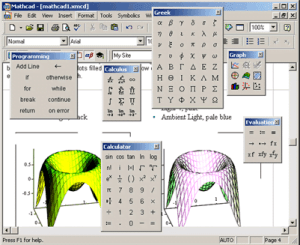## How to Know if MOSFET is Defective

Below are the guides on how to know if MOSFET is defective. These are the most common techniques that can be used to check if MOSFET is defective. Step #1 on How to Know if MOSFET is Defective :Diode Check The first thing we will try on how to know if MOSFET is defective is to check the diode drop.

## Protecting Devices from ESD Damage

In electronics industry, protecting devices from ESD damage is a must. Electrostatic discharge or simply ESD is a very high voltage spike that can easily damage small signal components such as integrated circuits and low power semiconductors. A common ESD is caused by human body touching to electronic devices. Charges are accumulating inside human body and then when any part## How to Calculate the Duty Cycle of Boost Converter

The inductor waveform is the key on how to calculate the duty cycle of boost converter. You can get direct equation for boost converter duty cycle formula from different sites but here I will discuss how it is derived. Meanwhile, a familiar boost converter schematic is shown in Figure 1. Figure 1 The inductor of the boost converter charges during## How to Saturate a PNP Transistor

PNP transistor is very useful in high side driving. A successful high side driving circuit starts with the proper understanding how to saturate a PNP transistor. Below are the steps to do it. How to Saturate a PNP Transistor – Step 1 Make sure that the circuit supplies are able to forward bias the emitter-base junction How to Saturate a## How to know if a Transistor is Saturated?

There are several ways how to know if a transistor is saturated or not. It is very important that if you design a transistor to function as a switch that it should operate in the saturation and cutoff. Operation at cutoff is simply interrupting the bias of the transistor. However operating at saturation is not that easy. You need to## Tools That Can be Used in Control System Design

Designing control systems needs a tool to speed up the task. Here are the tools that can be used in control system design. Matlab control systems toolbox is in the top of the list then followed by Simulink control design. I also included here simpowersystems because of its usefulness in power electronics. Control system is not as easy as ohms## How to Setup Bode Plot in Simulink

Below is the step by step procedure on how to setup bode plot in Simulink. Bode plot is a graph of gain and phase of a system. In a bode plot you can get the gain and phase margins and know if the system is stable or not. To demonstrate this properly, consider the Simulink model in Figure 1. You should## Monte Carlo Simulation using LTspice with Step by Step Tutorials

LTspice is a very good circuit simulation tool for electronic circuits. Thanks for Linear Technology for sharing such a nice tool. In this article I will teach Monte Carlo Simulation using LTspice with Step by Step Tutorials. Monte carlo is an analysis method used by design engineer to increase the robustness and reliability of their products. It is all about